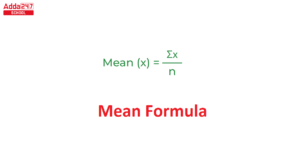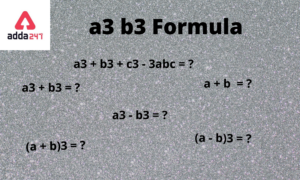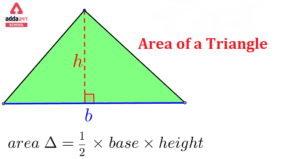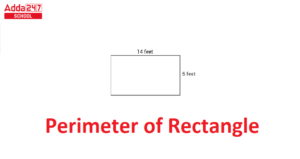Online Tution   »   Important Question   »   Heron's Formula

# Heron’s Formula, Proof and Example

## Heron’s Formula

Heron of Alexandria, also known as Hero, was a Greek geometer and inventor who lived around AD 62 in Alexandria, Egypt, and whose writings preserved knowledge of Babylonian, Egyptian, and Greco-Roman mathematics and engineering for posterity.

## Heron’s Formula for Area of Triangle

Metrica, Heron’s most important geometric work, was not discovered until 1896. It’s a three-volume compilation of geometric principles and formulas on areas and volumes of flat and solid forms that Heron compiled from a range of sources, some of which date back to ancient Babylon. The methods for calculating the area of various plane figures and the surface areas of common solids are listed in Volume I. A derivation of Heron’s (really, Archimedes’) formula for the area A of a triangle is included.

 A = √s(s−a)(s−b)(s−c)

where a, b, and c are the lengths of the sides of the triangle, and s is one-half the triangle’s perimeter or

 s = a + b +  c

Related Post:

## Heron’s Formula: Definition

Heron’s formula (also known as Hero’s formula) gives the area of a triangle given the lengths of all three sides are known in geometry. It is named after Hero of Alexandria, who is the person to find the formula. Unlike previous triangle area formulas, no angles or other distances in the triangle must be calculated first.

## Heron’s Formula: History

Heron of Alexandria penned Heron’s formula about 60 CE. He was a Greek engineer and mathematician who calculated the area of a triangle using only the lengths of its sides and went on to calculate the areas of quadrilaterals using the same method. This formula was used to prove trigonometric laws such as the Laws of Cosines and the Laws of Cotangents.

## Heron’s Formula: Example

Heron’s formula is a mathematical formula that may be used to calculate the area of a triangle given its three side lengths. It can be used to any shape or type of triangle as long as the three side lengths are known. Hero’s Formula is another name for it. Also, that it is not requited to know a triangle’s angle measurement to compute its area.

According to the Heron, the formula may be used to compute the area of any triangle, whether it is isosceles, equilateral, or scalene, given the sides of the triangle. Consider the triangle ABC, which has sides a, b, and c, respectively.

## Heron’s Formula in Hindi

हीरोन का सूत्र

अलेक्जेंड्रिया का बगुला, जिसे हीरो के रूप में भी जाना जाता है, एक ग्रीक जियोमीटर और आविष्कारक था, जो मिस्र के अलेक्जेंड्रिया में 62 ईस्वी के आसपास रहता था, और जिसके लेखन ने बेबीलोनियाई, मिस्र और ग्रीको-रोमन गणित और इंजीनियरिंग के ज्ञान को भावी पीढ़ी के लिए संरक्षित किया था।

त्रिभुज के क्षेत्रफल के लिए हीरोन का सूत्र

मेट्रिका, हेरॉन का सबसे महत्वपूर्ण ज्यामितीय कार्य, 1896 तक खोजा नहीं गया था। यह ज्यामितीय सिद्धांतों और फ़ार्मुलों का तीन-खंडों का संकलन है, जो समतल और ठोस रूपों के क्षेत्रों और संस्करणों पर आधारित है, जिसे हेरॉन ने कई स्रोतों से संकलित किया है, जिनमें से कुछ प्राचीन काल के हैं। बेबीलोन। विभिन्न समतल आकृतियों के क्षेत्रफल और सामान्य ठोसों के पृष्ठीय क्षेत्रफलों की गणना करने की विधियाँ खंड I में सूचीबद्ध हैं। त्रिभुज के क्षेत्रफल A के लिए हीरोन (वास्तव में, आर्किमिडीज़) के सूत्र की व्युत्पत्ति शामिल है।

ए = √s(s−a)(s−b)(s−c)

जहां a, b, और c त्रिभुज की भुजाओं की लंबाई हैं, और s त्रिभुज की परिधि का आधा है या

एस = ए + बी +  सी

## Heron’s Formula, Proof and Example- FAQs

Ques. What is Heron’s formula?

Ans. Heron’s Formula is used to calculate the area of a triangle. Area of a triangle using Heron’s Formula, A = √{s(s-a)(s-b)(s-c)}, in which a, b and c are the length of the three sides of a triangle and s being the semi-perimeter of the triangle, which is(a + b + c)/2.

Ques. Is Heron’s formula accurate?

Ans. Given the lengths of each side, Heron’s formula calculates the area of a triangle. A direct application of Heron’s formula may not be accurate if you have a very narrow triangle with two sides that are about equal and the third side is substantially shorter.

Ques. What is the formula of Semi perimeter?
Ans. The area of a scalene triangle is calculated using this formula. S (Semi perimeter) = (a + b + c)/2, where a, b, and c are the side lengths.

Ques. Can Heron’s formula be used for all triangles?

Ans. The Heron’s formula for the area of a triangle is applicable for all triangles and can be used to find the area of any triangle no matter whatever are the side lengths of the triangle.

Ques. Was Hero of Alexandria, Heron Greek?

Ans. Heron of Alexandria, also known as Hero, was a Greek geometer and inventor who lived around AD 62 in Alexandria, Egypt, and whose writings preserved knowledge of Babylonian, Egyptian, and Greco-Roman mathematics and engineering for posterity. Metrica, Heron’s most important geometric work, was not discovered until 1896.

Sharing is caring!

## FAQs

### What is Heron's formula?

Heron’s Formula is used to calculate the area of a triangle. Area of a triangle using Heron's Formula, A = √{s(s-a)(s-b)(s-c)}, in which a, b and c are the length of the three sides of a triangle and s being the semi-perimeter of the triangle, which is (a + b + c)/2.

### Is Heron's formula accurate?

Given the lengths of each side, Heron's formula calculates the area of a triangle. A direct application of Heron's formula may not be accurate if you have a very narrow triangle with two sides that are about equal and the third side is substantially shorter.

### What is the formula of Semi perimeter?

The area of a scalene triangle is calculated using this formula. S (Semi perimeter) = (a + b + c)/2, where a, b, and c are the side lengths.

### Can Heron's formula be used for all triangles?

The Heron's formula for the area of a triangle is applicable for all triangles and can be used to find the area of any triangle no matter whatever are the side lengths of the triangle.

### Was Hero of Alexandria, Heron Greek?

Heron of Alexandria, also known as Hero, was a Greek geometer and inventor who lived around AD 62 in Alexandria, Egypt, and whose writings preserved knowledge of Babylonian, Egyptian, and Greco-Roman mathematics and engineering for posterity. Metrica, Heron's most important geometric work, was not discovered until 1896.

### TOPICS:

•Water Cycle Diagram, Drawing for Kids of...
•Mean Formula and Meaning in Maths
•Decomposition Reaction, Examples, Formul...
•a3 b3 Formula- Proof, Solution and Examp...
•Area of Triangle- Formula in Class 10 Co...
•Perimeter of Rectangle Formula Unit, Que...